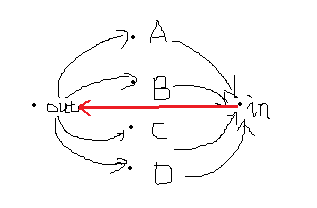# Meeting

Time Limit: 12000/6000 MS (Java/Others)    Memory Limit: 262144/262144 K (Java/Others)
Total Submission(s): 1112    Accepted Submission(s): 340

Problem Description
Bessie and her friend Elsie decide to have a meeting. However, after Farmer John decorated his
fences they were separated into different blocks. John's farm are divided into n blocks labelled from 1 to n.
Bessie lives in the first block while Elsie lives in the n-th one. They have a map of the farm
which shows that it takes they ti minutes to travel from a block in Ei to another block
in Ei where Ei (1im) is a set of blocks. They want to know how soon they can meet each other
and which block should be chosen to have the meeting.

Input
The first line contains an integer T (1T6), the number of test cases. Then T test cases
follow.

The first line of input contains n and m. 2n105. The following m lines describe the sets Ei (1im). Each line will contain two integers ti(1ti109) and Si (Si>0) firstly. Then Si integer follows which are the labels of blocks in Ei. It is guaranteed that mi=1Si106.

Output
For each test case, if they cannot have the meeting, then output "Evil John" (without quotes) in one line.

Otherwise, output two lines. The first line contains an integer, the time it takes for they to meet.
The second line contains the numbers of blocks where they meet. If there are multiple
optional blocks, output all of them in ascending order.

Sample Input
2 5 4 1 3 1 2 3 2 2 3 4 10 2 1 5 3 3 3 4 5 3 1 1 2 1 2

Sample Output
Case #1: 3 3 4 Case #2: Evil John
Hint
In the first case, it will take Bessie 1 minute travelling to the 3rd block, and it will take Elsie 3 minutes travelling to the 3rd block. It will take Bessie 3 minutes travelling to the 4th block, and it will take Elsie 3 minutes travelling to the 4th block. In the second case, it is impossible for them to meet.

Source

n m#include <stdio.h>
#include <string.h>
#include <iostream>
#include <algorithm>
#include <vector>
#include <queue>
#include <stack>
#include <set>
#include <map>
#include <string>
#include <math.h>
#include <stdlib.h>
#include <time.h>
using namespace std;
#define ll long long int
const int maxH = 3e6+10;
#define inf 999999999
struct Edge{
int to;
ll dis;
Edge(){};
Edge(int to_, ll dis_){to = to_, dis = dis_;}
bool operator < (const Edge& b)const{return dis>b.dis;}
}edge[maxH];
int head[maxH], tot, nxt[maxH];
ll A[maxH], B[maxH];
bool vis[maxH];
void add(int x, int y, ll dis){
edge[tot] = Edge(y, dis);
nxt[tot] = head[x];
head[x] = tot++;
}
void dijkstra(int st, ll f[]){
for(int i = 0;i <= maxH;i++) f[i] = inf;
priority_queue<Edge>q;
memset(vis, 0, sizeof vis);
f[st] = 0;
q.push(Edge(st, 0));
while(!q.empty()){
int x = q.top().to;
q.pop();
if(vis[x]) continue;
vis[x] = 1;
for(int i = head[x];i != -1;i = nxt[i]){
Edge e = edge[i];
//printf("%I64d %I64d %I64d\n", f[e.to], f[x], e.dis);
if(f[e.to] > f[x] + e.dis){
f[e.to] = f[x] + e.dis;
q.push(Edge(e.to, f[x] + e.dis));
}
}
}
}
int main(){
int T, n, m, t, s, k, e;
scanf("%d", &T);
k = 1;
while(T--){
tot = 1;
scanf("%d %d", &n, &m);
memset(head, -1, sizeof head);
for(int i = 1;i <= m;i++){
scanf("%d %d", &t, &s);
int in = n + i;
int out = n + m + i;
add(in, out, t);
while(s--){
scanf("%d", &e);
add(e, in, 0);
add(out, e, 0);
}
}
dijkstra(1, A);
dijkstra(n, B);
ll ans = inf;
int ed;
for(int i = 1;i <= n;i++){
ll tmp = max(A[i], B[i]);
//printf("%I64d %I64d\n", A[i], B[i]);
if(tmp <= ans){
ans = tmp;
ed = i;
}
}
printf("Case #%d: ", k++);
if(ans == inf) printf("Evil John\n");
else {
printf("%I64d\n", ans);
for(int i = 1;i < ed;i++){
if(max(A[i], B[i]) == ans)
printf("%d ", i);
}
printf("%d\n", ed);
}
}
}